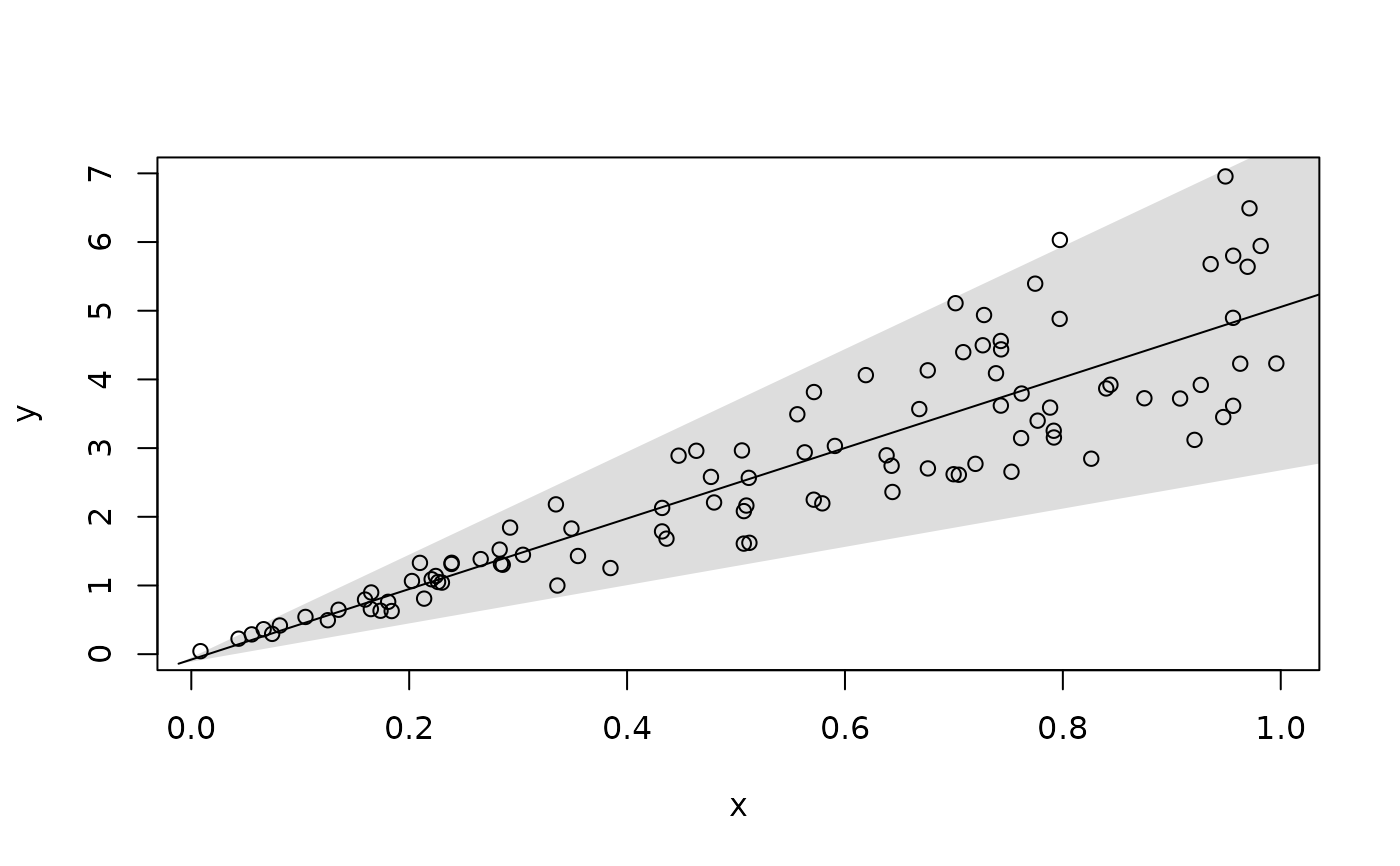Produce a linear, quadratic, or nonparametric tube for regression data.

## Usage

makeTube(
x,
y,
Z = 2,
R = 1,
col = "#00000022",
border = "#00000000",
stDev = c("constant", "linear", "other"),
length.out = 99,
bw = "default",
plotTube = TRUE,
...
)

## Arguments

x

x coordinates.

y

y coordinates.

Z

Number of standard deviations out from the regression line to extend the tube.

R

Control of how far the tube extends to the left and right.

col

Fill color of the tube.

border

Border color of the tube.

type

The type of model fit to the data. Here 'robust' results in a nonparametric estimate.

stDev

Choices are constant variance ('constant'), the standard deviation of the errors changes linearly ('linear'), or the standard deviation of the errors should be estimated using nonparametric methods ('other').

length.out

The number of observations used to build the regression model. This argument may be increased to increase the smoothing of a quadratic or nonparametric curve.

bw

Bandwidth used if type='robust' or homosk=FALSE.

plotTube

Whether the tube should be plotted.

Whether the linear model should be plotted.

...

Additional arguments passed to the lines function if addLine=TRUE.

## Value

X

x coordinates for the regression model.

Y

y coordinates for the regression model.

tubeX

x coordinates for the boundary of the tube.

tubeY

y coordinates for the boundary of the tube.

lmPlot

David Diez

## Examples


# possum example
plot(possum$total_l, possum$head_l)
makeTube(possum$total_l, possum$head_l, 1)
makeTube(possum$total_l, possum$head_l, 2)
makeTube(possum$total_l, possum$head_l, 3)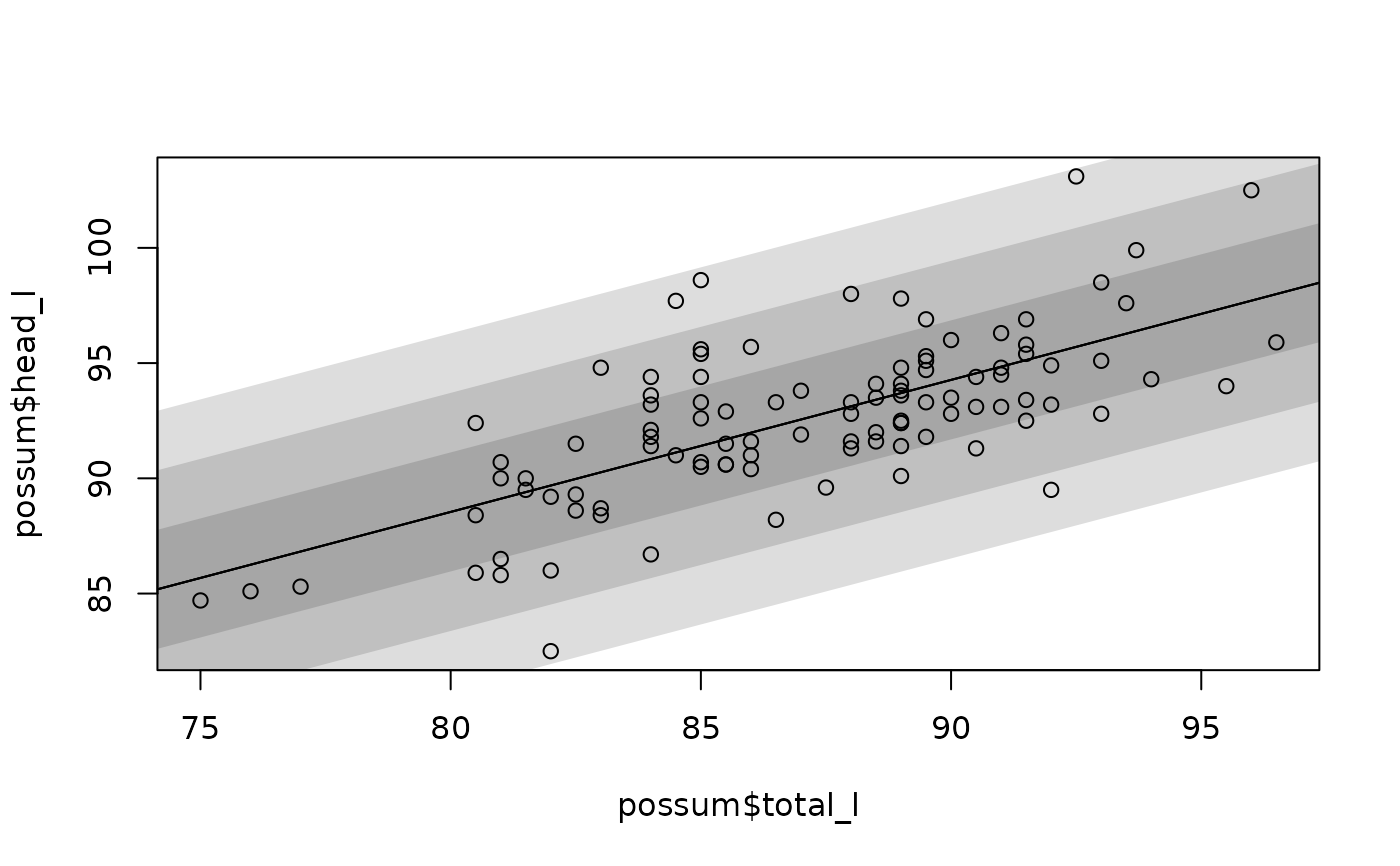makeTube(gradestv$tv, gradestv$grades, 1.5)makeTube(gradestv$tv, gradestv$grades, 1.5, stDev = "o")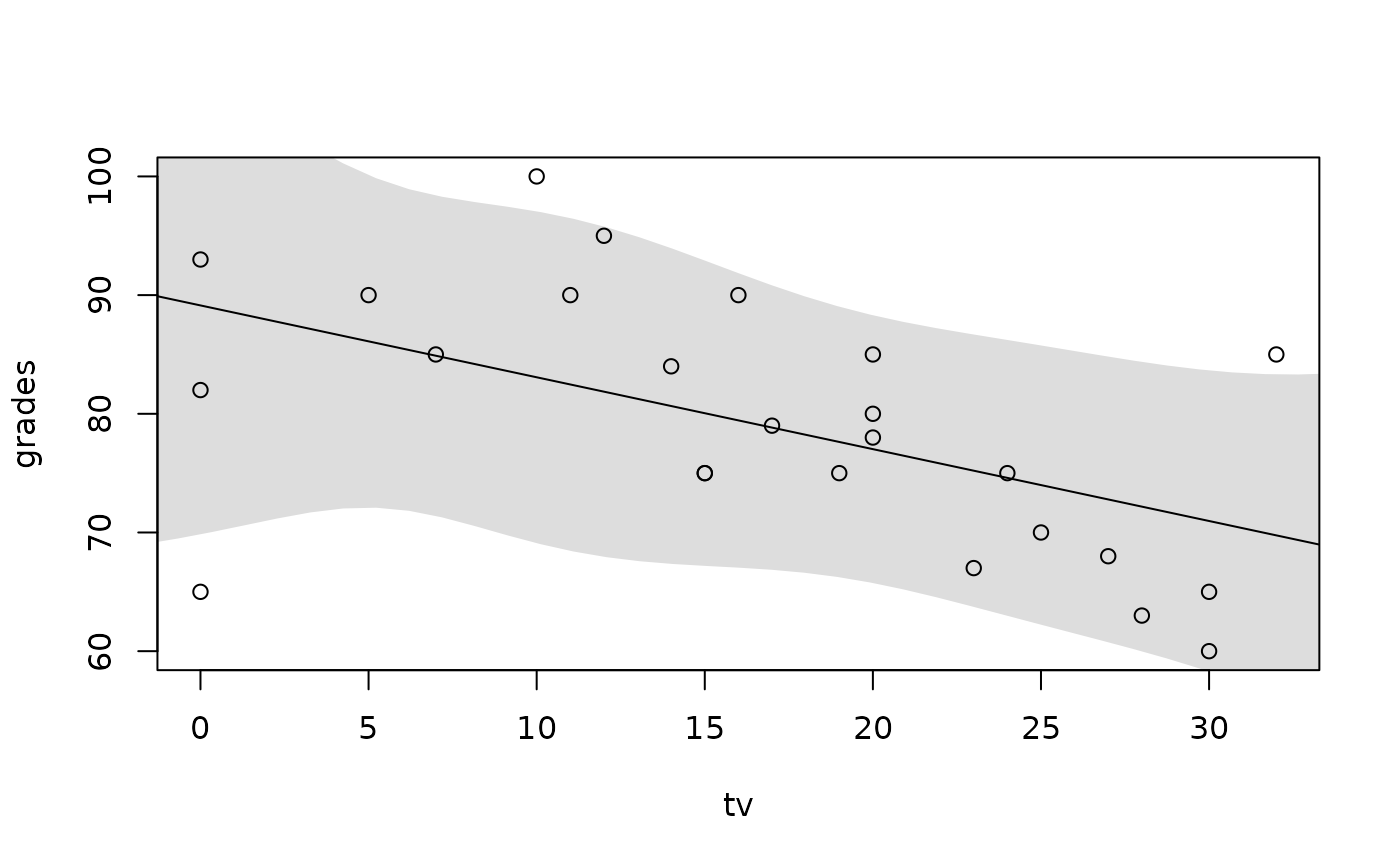makeTube(gradestv$tv, gradestv$grades, 1.5, type = "robust")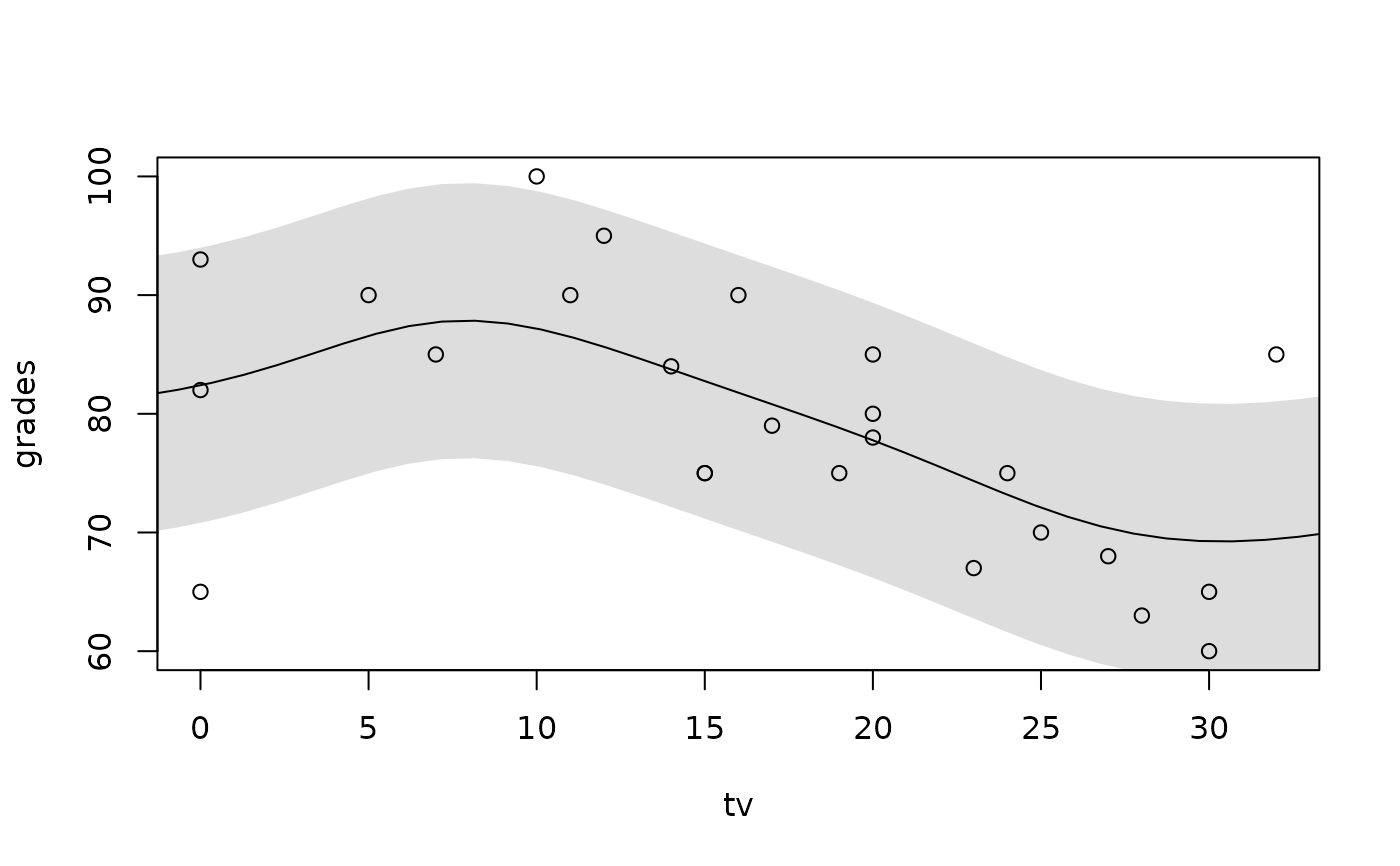makeTube(gradestv$tv, gradestv$grades, 1.5, type = "robust", stDev = "o")# what can go wrong with a basic least squares model
# 1
x <- runif(100)
y <- 25 * x - 20 * x^2 + rnorm(length(x), sd = 1.5)
plot(x, y)
makeTube(x, y, type = "q")# 2
x <- c(-0.6, -0.46, -0.091, runif(97))
y <- 25 * x + rnorm(length(x))
y <- y + 8
y <- y + 1
plot(x, y, ylim = range(y) + c(-10, 5))
makeTube(x, y)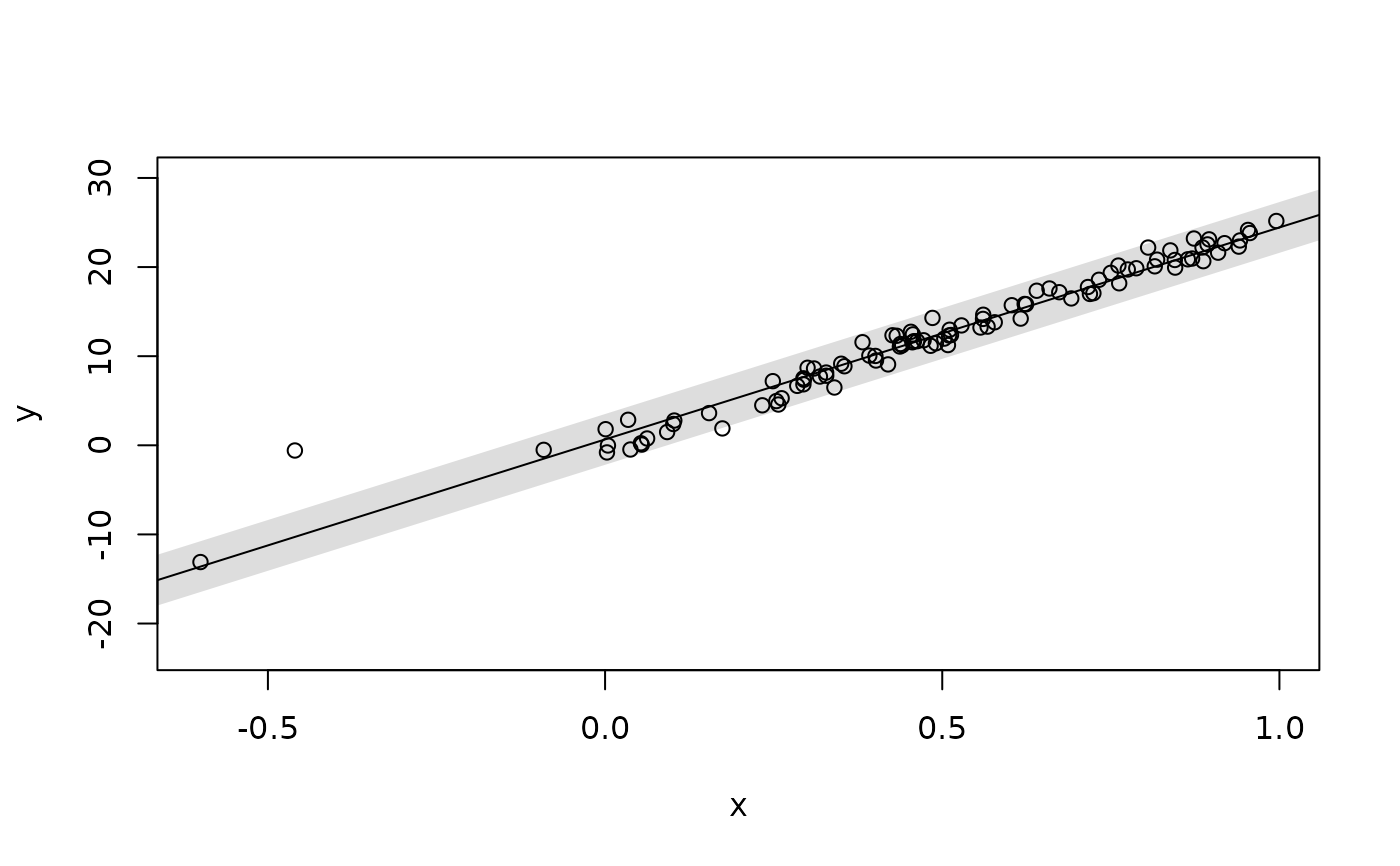# 3
x <- runif(100)
y <- 5 * x + rnorm(length(x), sd = x)
plot(x, y)
makeTube(x, y, stDev = "l", bw = 0.03)